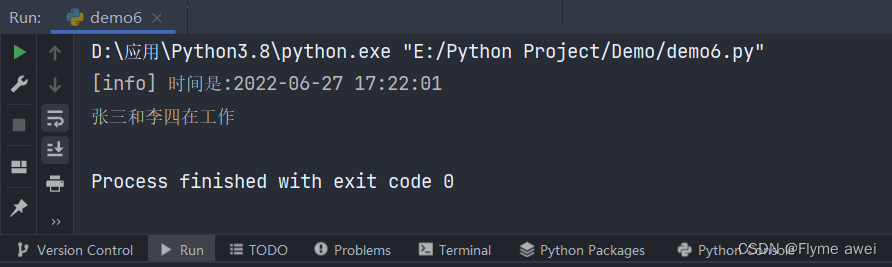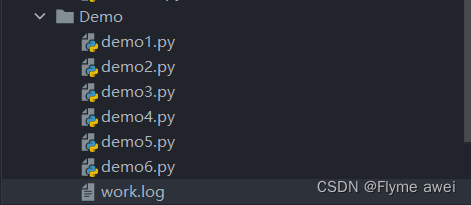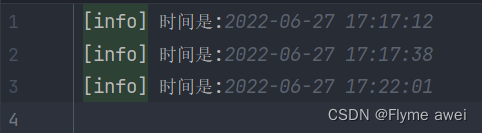> 文档中心 > 【Python函数式编程⑤】——返回函数、闭包、装饰器、偏函数

# 【Python函数式编程⑤】——返回函数、闭包、装饰器、偏函数🐚 作者: 阿伟
💂 个人主页: Flyme awei

🐋 希望大家多多支持😘一起进步呀！

💬 文章对你有帮助👉关注✨点赞👍收藏📂

# Python 高级编程### 目录

• Python 高级编程
• 一、返回函数
• 二、闭包
• 三、装饰器 wraps
• 3.1什么是装饰器
• 3.2带参装饰器
• 3.3函数做装饰器
• 3.4类做装饰器
• 四、偏函数 partial

# 一、返回函数

``# -*- coding: utf-8 -*-# python 全栈# author : a wei # 开发时间: 2022/6/23 22:41def sum_fun_a(*args):    a = 0    for n in args: a = a + n   return a``

``# -*- coding: utf-8 -*-# python 全栈# author : a wei # 开发时间: 2022/6/23 22:41def sum_fun_b(*args):    def sum_a(): a = 0 for n in args:     a = a + n return a    return sum_a``

``f1 = sum_fun_b(1, 2, 3, 4, 5)#  此时f为一个对象实例化，并不会直接生成值print(f1())  # 15f2 = sum_fun_b(1, 2, 3, 4, 5)f3 = sum_fun_b(1, 2, 3, 4, 5)print(f2, f3)<function sum_fun_b.<locals>.sum_a at 0x0000016E1E1EFD30> <function sum_fun_b.<locals>.sum_a at 0x0000016E1E1EF700>print(id(f2), id(f3))1899067537152 1899067538880``

# 二、闭包

``# 定义一个函数def fun_a(num_a):# 在函数内部再定义⼀个函数# 并且这个内部函数⽤到了外部的变量，这个函数以及⽤到外部函数的变量及参数叫 闭包    def fun_b(num_b): print('内嵌函数fun_b的参数是:%s,外部函数fun_a的参数是:%s' % (num_b, num_a)) return num_a + num_b # 这里返回的就是闭包的结果    return fun_b# 给fun_a函数赋值，这个10就是传参给fun_aret = fun_a(10)# 注意这里的10其实是赋值给fun_bprint(ret(10))# 注意这里的90其实是赋值给fun_bprint(ret(90))``

``内嵌函数fun_b的参数是:10,外部函数fun_a的参数是:1020内嵌函数fun_b的参数是:90,外部函数fun_a的参数是:10100``

``"""三个条件，缺一不可： 1)必须有一个内嵌函数(函数里定义的函数）——这对应函数之间的嵌套 2)内嵌函数必须引用一个定义在闭合范围内(外部函数里)的变量——内部函数引用外部变量3)外部函数必须返回内嵌函数——必须返回那个内部函数"""``

`````python# python交互环境编辑器 >>> def counter(start=0): count = [start]def incr(): count += 1 return count return incr >>> c1 = counter(5)>>> print(c1()) 6>>> print(c1()) 7>>> c2=counter(50) >>> print(c2()) 51>>> print(c2()) >52>>>``

``def test():  count = 1  def add():   print(count)   count += 1  return add a = test() a()``

``Traceback (most recent call last): ...... UnboundLocalError: local variable 'count' referenced before assignment``

``# -*- coding: UTF-8 -*- # def test():  # count不是局部变量，介于全局变量和局部变量之间的一种变量，nonlocal标识 count = 1  def add():   nonlocal count   print(count)   count += 1   return count  return add a = test() a() # 1 a() # 2``

nonlocal声明的变量不是局部变量,也不是全局变量,而是外部嵌套函数内的变量。

``1. 每次调用函数时，都得在全局作用域申明变量。别人调用函数时还得查看函数内部代码。 3. 当函数在多个地方被调用并且同时记录着很多状态时，会造成非常地混乱。``

``def line_conf(a, b): def line(x): return a * x + b return lineline1 = line_conf(1, 1) line2 = line_conf(4, 5) print(line1(5)) print(line2(5))``

``625``

``1.闭包似优化了变量，原来需要类对象完成的⼯作，闭包也可以完成 2.由于闭包引⽤了外部函数的局部变量，则外部函数的局部变量没有及时释放，消耗内存``

``def fun_a(): fun_list = [] for i in range(1, 4): def fun_b(): return i * i fun_list.append(fun_b) return fun_list f1, f2, f3 = fun_a() print(f1(), f2(), f3())# 结果：9，9，9``

``def test3():    func_list = []    for i in range(1, 4): def test4(i_= i):     return i_**2 func_list.append(test4)    return func_listf1, f2, f3 = test3()print(f1(), f2(), f3())``

``# -*- coding: UTF-8 -*- # def fun_a(): def fun_c(i): def fun_b(): return i * i return fun_b fun_list = [] for i in range(1, 4): # f(i)立刻被执行，因此i的当前值被传入f() fun_list.append(fun_c(i)) return fun_list f1, f2, f3 = fun_a() print(f1(), f2(), f3()) # 1 4 9``

# 三、装饰器 wraps

``# -*- coding: utf-8 -*-# python 全栈# author : a wei # 开发时间: 2022/6/24 17:03def eat():    print('吃饭')def test1(func):    def test2(): print('做饭')  func()  print('洗碗')    return test2eat()  # 调用eat函数# 吃饭test1(eat)()# 做饭# 吃饭# 洗碗``

## 3.1什么是装饰器

``"""装饰器本质上是一个Python函数，它可以让其他函数在不需要做任何代码变动的前提下增加额外功能，装饰器的返回值 也是一个函数对象。 它经常用于有以下场景，比如：插入日志、性能测试、事务处理、缓存、权限校验等场景。装饰器是解决这类问题的绝 佳设计"""``

``# -*- coding: utf-8 -*-# python 全栈# author : a wei # 开发时间: 2022/6/24 17:03def test1(func):    def test2(): print('做饭') func() print('洗碗')    return test2@test1  # 装饰器def eat():    print('吃饭')eat()# 做饭# 吃饭# 洗碗``

(注意：@在装饰器这里是作为Python语法里面的语法糖写法，用来做修饰。)

``print(eat.__name__)# test2``

`` -*- coding: utf-8 -*- from functools import wrapsdef test1(func):    @wraps(func)    def test2(): print('做饭') func() print('洗碗')    return test2@test1  # 装饰器def eat():    print('吃饭')eat()# 做饭# 吃饭# 洗碗print(eat.__name__)# eat``

``# -*- coding: utf-8 -*-# python 全栈# author : a wei # 开发时间: 2022/6/24 17:42import timefrom functools import wrapsdef logger(func):    @wraps(func)    def write_log(): print('[info]--时间:%s' % time.strftime('%Y-%m-%d %H:%M:%S')) func()    return write_log@loggerdef work():    print('我在工作')work()# [info]--时间:2022-06-24 17:52:11# 我在工作print(work.__name__)#work``

## 3.2带参装饰器

``# -*- coding: utf-8 -*-# python 全栈# author : a wei # 开发时间: 2022/6/24 17:42import timefrom functools import wrapsdef logs(func):    @wraps(func)    def write_log(*args, **kwargs): print('[info]--时间:%s' % time.strftime('%Y-%m-%d %H:%M:%S')) func(*args, **kwargs)    return write_log@logsdef work():    print('我在工作')@logsdef work2(name1, name2):    print('%s和%s在工作' % (name1, name2))work2('张三', '李四')# [info]--时间:2022-06-24 18:04:04# 张三和李四在工作``

## 3.3函数做装饰器

``# -*- coding: utf-8 -*-# python 全栈# author : a wei# 开发时间: 2022/6/20 0:06import timefrom functools import wrapsdef logger(file):    def logs(fun): @wraps(fun) def write_log(*args, **kwargs):     log = '[info] 时间是:%s' % time.strftime('%Y-%m-%d %H:%M:%S')     print(log)     with open(file, 'a+') as f:  f.write(log)     fun(*args, **kwargs) return write_log    return logs@logger('work.log')  # 使用装饰器来给 work函数增加记录日志的功能def work(name, name2):  # 1.当前 work可能有多个参数 2.自定义日志文件的名字和位置，记录日志级别    print(f'{name}和{name2}在工作')work('张三', '李四')``## 3.4类做装饰器

``# -*- coding: utf-8 -*-# python 全栈# author : a wei# 开发时间: 2022/6/20 0:06import timefrom functools import wraps# 不使用函数做装饰器，使用类做装饰器class Logs(object):    def __init__(self, log_file='out.log', level='info'): # 初始化一个默认文件和默认日志级别 self.log_file = log_file self.level = level    def __call__(self, fun):  # 定义装饰器，需要一个接受函数 @wraps(fun) def write_log(name, name2):     log = '[%s] 时间是:%s' % (self.level, time.strftime('%Y-%m-%d %H:%M:%S'))     print(log)     with open(self.log_file, 'a+') as f:  f.write(log)     fun(name, name2) return write_log@Logs()  # 使用装饰器来给 work函数增加记录日志的功能def work(name, name2):  # 1.当前 work可能有多个参数 2.自定义日志文件的名字和位置，记录日志级别    print(f'{name}和{name2}在工作')work('张三', '李四')  # 调用work函数``

# 四、偏函数 partial

Python的 functools 模块提供了很多有用的功能，其中一个就是偏函（Partial function）。要注意，这里的偏函数和数学意义上的偏函数不一样。

``>>> int('123') 123``

``>>> int('12345', base=8) 5349 >>> int('12345', 16) 74565``

``# 定一个转换义函数 >>> def int_1(num, base=2): return int(num, base) >>> int_1('1000000') 64>>> int_1('1010101') 85``

``# 导入 >>> import functools # 偏函数处理 >>> int_2 = functools.partial(int, base=2) >>> int_2('1000000') 64>>> int_2('1010101') 85``

``int2('10010')``

``kw = { base: 2 } int('10010', **kw)``